Choose whether the given statement is true or false.The sides of a triangle have lengths 11,15 and k where k is an integer. Thus, is the values of k and it is the triangle obtuse?

# Choose whether the given statement is true or false.The sides of a triangle have lengths 11,15 and k where k is an integer. Thus,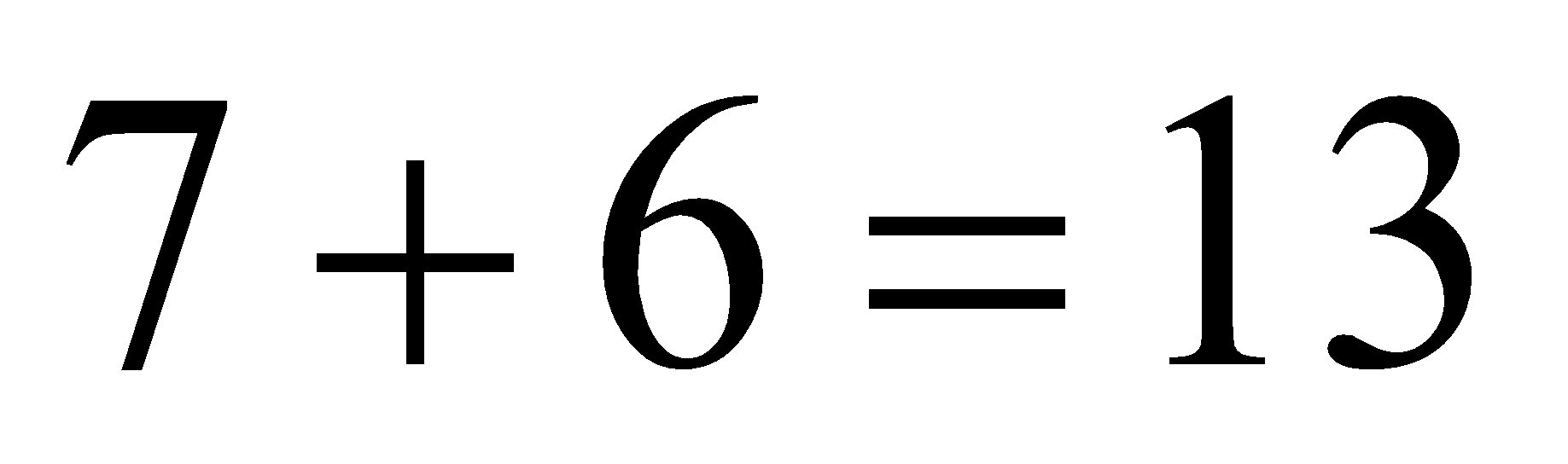is the values of k and it is the triangle obtuse?

1. A
True
2. B
False

Fill Out the Form for Expert Academic Guidance!l

+91

Live ClassesBooksTest SeriesSelf Learning

Verify OTP Code (required)

### Solution:

Given: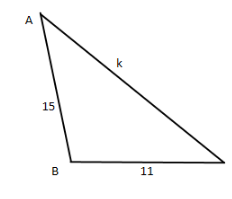Here, Sum of two sides of triangle is more than third side,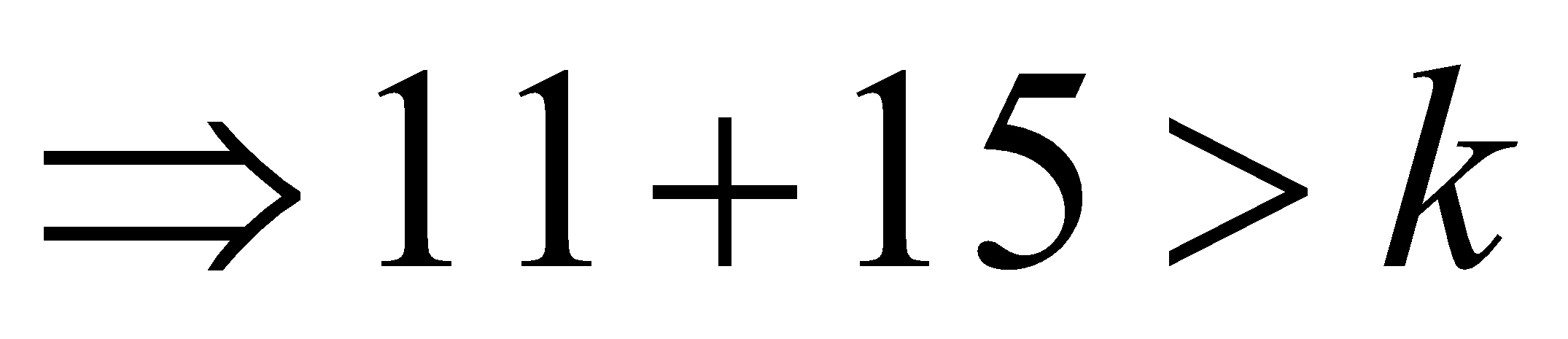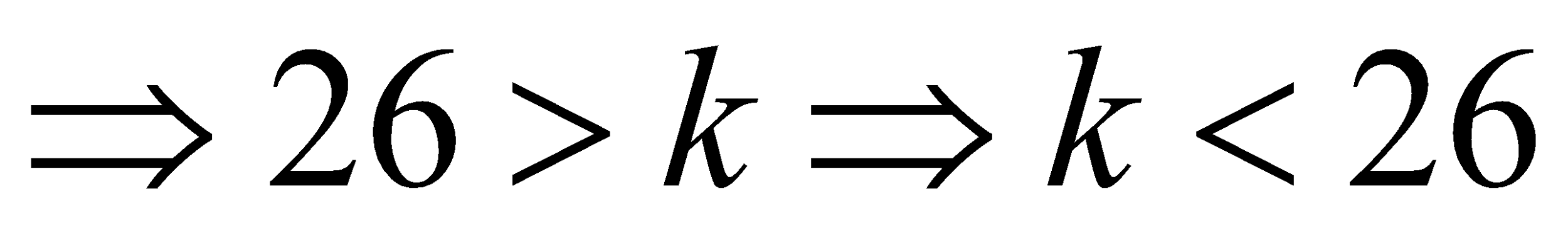The difference of any two sides of triangle is less than third side,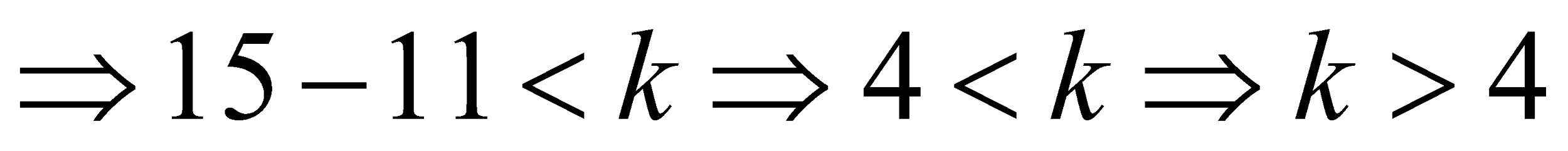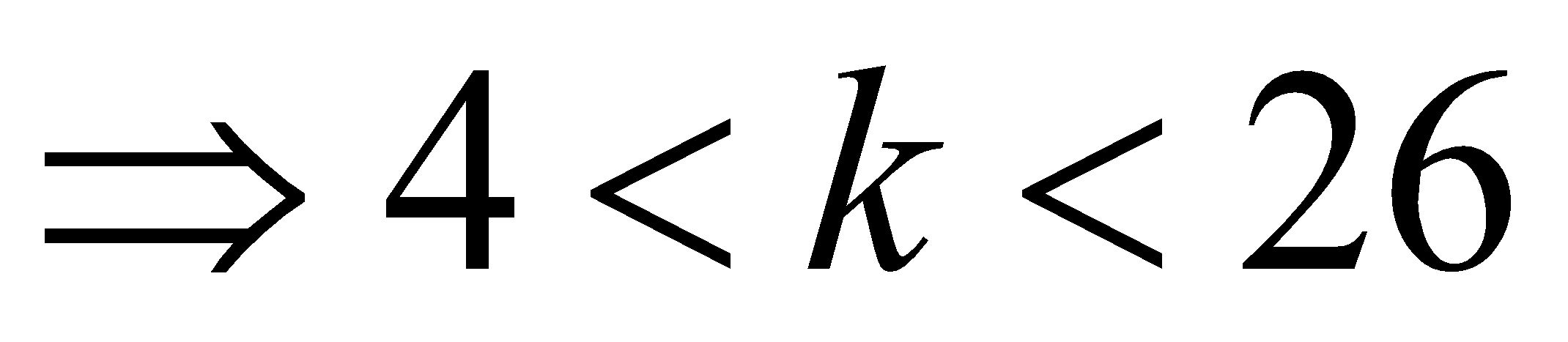Thus,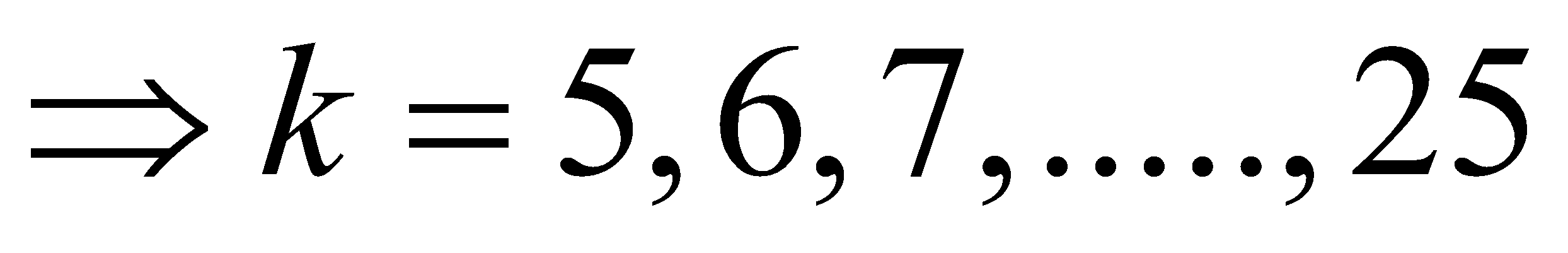A triangle is said to be obtuse; the square of a side should be greater than the sum of squares of the other two sides.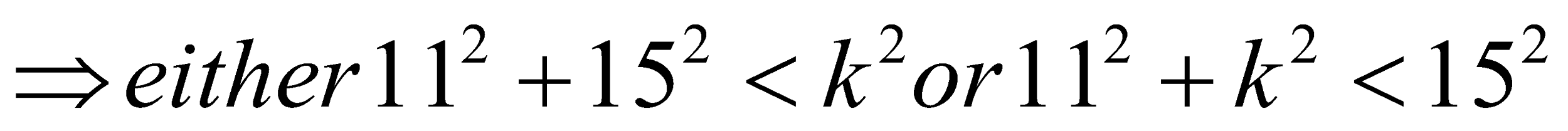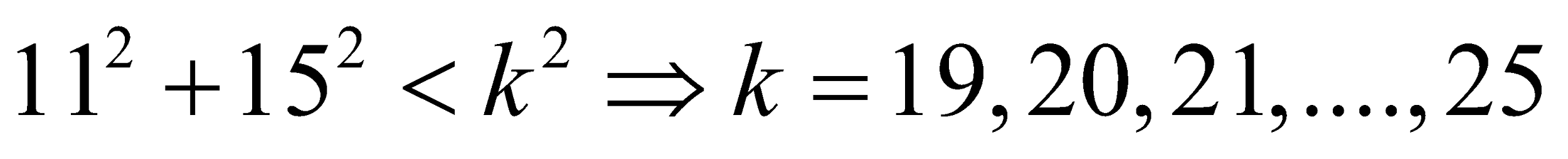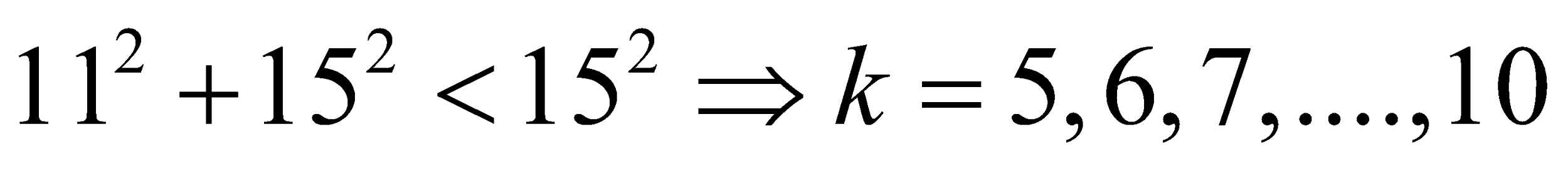Number of values possible for k are:Hence, the given statement is true.

## Related content

 Area of Square Area of Isosceles Triangle Pythagoras Theorem Triangle Formula Perimeter of Triangle Formula Area Formulae Volume of Cone Formula Matrices and Determinants_mathematics Critical Points Solved Examples Type of relations_mathematics+91

Live ClassesBooksTest SeriesSelf Learning

Verify OTP Code (required)